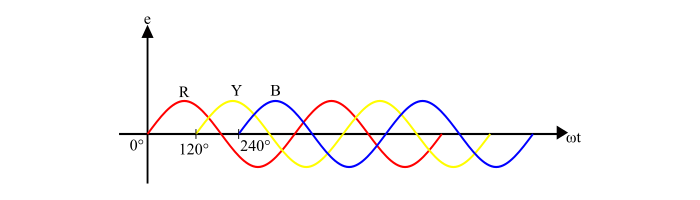# Basics of Three-Phase Electricity

ElectronElectronics & ElectricalDigital Electronics

The phase-electricity is produced by a 3-phase generator that has three separate but identical windings, which are called phases. The 3-phase windings of the 3-phase generator are displaced 120° apart from each other and rotate in a common uniform magnetic field. Therefore, a three-phase generator produces three voltages of the same magnitude and frequency but 120° electrical apart from one another.Consider a three-phase alternator as shown in the figure. It has three identical windings or coils R, Y and B displaced 120° electrical from each other and rotating in anticlockwise direction with an angular velocity ω in a magnetic field create by the two pole magnet. Since, the three coils are identical and have the same angular velocity, the emf induced in them will be of the same magnitude and frequency. Although, the three emf will be displaced from one another by 120° electrical.From the wave diagram, it can be seen that the emf in coil Y being 120° behind that of coil R and the emf in coil B being 240° behind that of coil R. The equations of three emf can be given as −

$$E_{R}=E_{m}\sin\omega\:t$$

$$E_{Y}=E_{m}\sin(\omega\:t-120^{\circ})$$

$$E_{B}=E_{m}\sin(\omega\:t-240^{\circ})$$

## Three-Phase System Terminology

• Phase Sequence – The phase sequence is defined as the order in which the emf in three phase or coils of an alternator attains the positive maximum value. It is determined by the direction of rotation of alternator.

• Naming the Phases – The name of the three phases are given by the natural colors viz. Red (R), Yellow (Y) and Blue (B). Thus, the phase sequence being RYB.

• Balanced Three-Phase Supply System – In a balanced three-phase supply system, the three phase voltages are equal in magnitude and frequency.

## Connections of Three-Phases

In practice, the three phase windings of a three-phase alternator is connected in two ways −

• Star or Wye Connection – In star connection, the similar ends (start or finish) of the three-phases are connected together within the alternator and three lines are taken out from the other three free ends (see the figure). A neutral wire may or may not be brought out. If the neutral is present then the system is called three-phase four-wire system whereas if no neutral is taken, the system is called 3-phase 3-wire system.• Delta Connection – In delta connection, dissimilar ends (start to finish) of three windings are connected together and three lines are run from the junction points (see the figure). In delta connection, neutral point does not exists. Therefore, only 3-phase 3-wire system can be formed.## Reasons for the use of Three-Phase Electricity

• Three-phase power has constant magnitude whereas single phase being the function of frequency.

• A three-phase system can setup a rotating magnetic field (RMF) in stationary windings of 3-phase electrical machines.

• For the same rating, three-phase machines have smaller and simpler construction.

• To transmit same amount of power a three-phase system requires less copper material as compared to single phase system.

• The three-phase transmission lines have better voltage regulation.